### MEMO 2018 ekipno problem 7

Kvaliteta:
Avg: 0,0
Težina:
Avg: 6,0

Let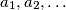$a_1, a_2, \ldots$ be a sequence of positive integers such that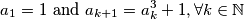Prove that for every prime number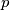$p$ of the form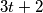$3t+2$, where$t$ is a non-negative integer, there exists a positive integer$n$ such that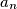$a_n$ is divisible by$p$.

Izvor: Srednjoeuropska matematička olimpijada 2018, ekipno natjecanje, problem 7